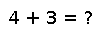# permutedims

permutedims(A, perm)

Permute the dimensions of array `A`. `perm` is a vector specifying a permutation of length `ndims(A)`. This is a generalization of transpose for multi-dimensional arrays. Transpose is equivalent to `permutedims(A, [2,1])`.

## Examples

Checking you are not a robot: